# Symbolic Analysis Workshop @ FoCM, Hong Kong

http://www-sop.inria.fr/cafe/SA08

June 24-26, 2008

Schedule

(pdf)

 Tuesday 24th Wednesday 25th Thursday 26th 13:50-14:30 Moulay Barkatou Min Wu* Markus Rosenkranz 14:40-15:20 Peter van der Kamp George Labahn / Michael Singer Elizabeth Mansfield 15:30-16:00 coffee break coffee break coffee break 16:00-16:40 Peter Paule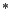José Cano Peter Olver 16:50-17:30 Raimundas Vidunas Ekaterina Shemyakova Hiroshi Umemura17:40-18:20 Xiao-Shan Gao, Chunming Yuan Mark Hickman Ziming Li

Hypergeometric Solutions of Systems of Linear Difference Equations and Applications

M. A. Barkatou

XLIM Institute, Université de Limoges, France
moulay.barkatou@xlim.fr

In this talk we present a new algorithm for computing hypergeometric solutions of systems of linear difference equations and discuss applications to finding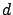'th order (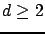) right-hand factors of a given linear difference operator with polynomial coefficients.

This talk is based on a joint work with Mark van Hoeij.

Formal power series solutions of Ordinary Differential Equations and the Newton polygon method.

Jose Cano

jcano@agt.uva.es
The Newton polygon method has proved useful for finding power series (with real exponents) solutions of differential equations. The method is an extension by Briot and Bouquet (1856) and Fine (1889) of the polygon construction used by Puiseux in his studies of algebraic curves. Despite its elementary nature, it has been used for proving some remarkable results we shall review, with special emphasis in a recent one: that the set of truncations of generalized power series solutions of an ODE is contained in a semi-algebraic set whose dimension is bounded by two times the order of the equation.

Characteristic Set Method for Differential-Difference Polynomial Systems

Xiao-Shan Gao, Chumin Yuan

Key Laboratory of Mathematics Mechanization, AMSS, Chinese Academy of Sciences, Beijing, China
xgao@mmrc.iss.ac.cn
In this talk, we present a characteristic set method for mixed differential and difference polynomial systems. We will introduce the concepts of coherent, regular, proper irreducible, and strongly irreducible ascending chains and their properties. We give an algorithm which can be used to decompose the zero set for a finitely generated differential and difference polynomial set into the union of the zero sets of regular and consistent ascending chains. As a consequence, we give an algorithm to solve the perfect ideal membership problem for differential and difference polynomials.

Leading Order Integrability Conditions for Differential-Difference Equations

Mark Hickman

Department of Mathematics & Statistics University of Canterbury, New Zealand
M.Hickman@math.canterbury.ac.nz
A necessary condition for the existence of conserved densities, r, and fluxes of an autonomous differential-difference equation which depend on q-shifts, for q sufficiently large, is presented. The condition depends on the eigenvalues of the leading terms in the differential-difference equation. It also gives, explicitly, the leading integrability conditions on the density in terms of second derivatives of r. This analysis has led to the development of an algorithm for computing density/flux pairs for such equations. For a scalar equation, the algorithm has been coded in MAPLE . It is more efficient (in both storage and speed) that existing algorithms.

Symmetries of PDEs, and integrability

Peter van der Kamp

Mathematics, La Trobe University, Victoria, Australia.
peterhvanderkamp@gmail.com
In the majority of cases where exact solutions of differential equations can be found, the underlying property is a (continuous) symmetry of the equation. And, in the theory of integrable equations, the recognition and classification methods based on the existence of symmetries have been particular successful.

A symmetry-group transforms one solution of an equation to another solution of the same equation. This idea goes back to Sophus Lie. Olver writes: The great power of Lie group theory lies in the crucial observation that one can replace the complicated, nonlinear conditions for the invariance of the solution set of an equation under the group transformations by an equivalent linear condition of infinitesimal invariance under the corresponding infinitesimal generators of the group action.

My talk consists of two parts. Firstly, I will provide an (infinitesimal) characterization of symmetries that is different from the standard one, generalizing a similar characterization in the special settings of ODEs and evolution equations to the setting of passive orthonomic systems. And secondly, I will review some results on the symmetry-classification of integrable evolution equations.

Popov Forms of Matrices of Differential Polynomials

George Labahn

D. R. Cheriton School of Computer Science, University of Waterloo, Ontario, Canada
glabahn@cs.uwaterloo.ca
Popov normal forms were introduced by V. Popov for matrices of polynomials in the middle 1960s as an alternative to the better known Hermite normal form. They were found to have better properties for use in the context of linear control theory. In this talk we will discuss Popov normal forms for matrices of differential operators. We show their usefulness in the context of systems of linear differential equations and discuss the various computational challenges in computing the forms for arbitrary matrices of differential operators.

Simplifying Skew Fractions Modulo Quasi-Linear Difference Relations

Ziming Li

Key Laboratory of Mathematics Mechanization, AMSS, Chinese Academy of Sciences, Beijing, China
zmli@mmrc.iss.ac.cn
This work is motivated by simplifying transfer functions (matrices) of nonlinear discrete-time input/output systems in control theory.

Let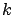be a field of characteristic zero, and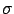an automorphism of. Consider a first-order quasi-linear system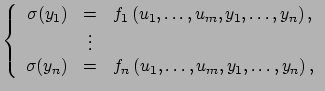where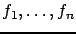are rational functions. We present a criterion that determines whether the field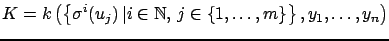is difference field with the induced monomorphism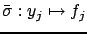,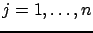. We construct its inversive closure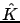when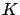is a difference field.

Let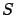be the skew polynomial ring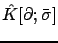. We describe a few approaches to computing the greatest common left divisors (gcld's) of two polynomials in. Every element in the left-fraction field ofcan be simplified by removing the gcld of its numerator and denominator.

The transfer function for a discrete-time single-input single-output equation is an element of the left-fraction field of, while the transfer matrix for a discrete-time multiple-input multiple-output system has entries in the same skew-field. The goal of our investigation is to develop efficient algorithms for manipulating transfer functions (matrices).

This talk is based on joint work with Martin Ondera, and Huaifu Wang.

Elizabeth L. Mansfield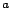and G. Reinout W. Quispel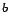University of Kent, UKLa Trobe University, Australia
E.L.Mansfield@kent.ac.uk

Discrete gradients approximate the flow of a gradient vector field and have several important properties that make them interesting to geometric integrators. In particular, when used with a symplectic form to approximate a hamiltonian flow, exact conservation of integrals may be obtained.

In this talk we discuss the definition and applications of discrete gradients, and show how they relate to symbolically determined function spaces defined in terms of moments. In particular we show how an infinite set of discrete gradients may be obtained, exactly in a symbolic computation environment, for a given function.

The talk concludes with a discussion of on-going work and open problems.

The authors wish to thank the Institute of Advanced Studies, LaTrobe University and the Australian Research Council.

Invariant Variational Problems and Invariant Flows

Peter J. Olver

School of Mathematics, University of Minnesota, Minneapolis, USA
olver@umn.edu
I will present the moving frame approach to the analysis of invariant variational problems and the evolution of differential invariants under invariant submanifold flows. Applications will include differential geometric flows, solitons and Poisson structures, and computer vision.

Symbolic Analysis in Combinatorics and Special Functions

Peter Paule

Research Institute for Symbolic Computation, Johannes Kepler University, Linz, Austria
ppaule@risc.uni-linz.ac.at
The talk presents a variety of applications of symbolic analysis: orthogonal polynomials (e.g. positivity and log-concavity of coefficients), special function inequalities, definite integrals from physics, hypergeometric series, and combinatorial (multiple) sums. In all these examples I illustrate the usage of computer algebra software developed in my group at RISC.

Integro-Differential Polynomials and Boundary Problems

Markus Rosenkranz, Georg Regensburger

Radon Institute for Computational and Applied Mathematics, Austrian Academy of Sciences, Linz
Markus.Rosenkranz@oeaw.ac.at
While differential polynomials can be used for formally adjoining solutions of nonlinear ordinary differential equations (and systems), their initial or boundary conditions are typically ignored. We introduce a new algebraic structure that tries to remedy this flaw: The integro-differential polynomials can be seen as a generalization of differential polynomials that includes integral operators and the corresponding evaluations. By incorporating the Baxter rule (integration by parts''), they also contain the shuffle algebra.

Our approach is constructive by using a suitable system of canonical forms. The result is an integro-differential algebra: one can add, multiply, differentiate, integrate, and evaluate integro-differential polynomials. Formal solutions can be adjoined by factoring out the (integro-)differential equation and its initial conditions.

Moving Frames for Laplace Invariants

Ekaterina Shemyakova

RISC, Hagenberg, Austria.
kath@risc.uni-linz.ac.at
The development of symbolic methods for the factorization and integration of linear PDEs, many of the methods being generalizations of the Laplace transformations method, requires the finding of complete generating sets of invariants for the corresponding linear operators and their systems with respect to the gauge transformations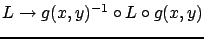. Within the theory of Laplace-like methods, there is no uniform approach to this problem, though some individual invariants for hyperbolic bivariate operators, and complete generating sets of invariants for second- and third-order hyperbolic bivariate ones have been obtained.

We show a systematic and much more efficient approach to the same problem by application of moving-frame methods. We give explicit formulae for complete generating sets of invariants for second- and third-order bivariate linear operators, hyperbolic and non-hyperbolic, and also demonstrate the approach for pairs of operators appearing in Darboux transformations.

Differential Groups and the Gamma Function

Michael Singer

Department of Mathematics, North Carolina State Univerity, Raleigh, USA.
singer@math.ncsu.edu
I will present a Galois theory of linear difference equations where the Galois group are linear differential groups that is, groups of matrices whose entries satisfy a fixed set of polynomial differential equations. These groups measure the differential dependence among solutions of linear difference equations.

I will give a general introduction to the theory of linear differential groups anddiscuss how this theory can be used to reprove Hölder's Theorem that the Gamma function satisfies no differential polynomial equation as well as new results concerning differential dependence of solutions of higher order difference equations, such as families of q-hypergeometric equations.

This is joint work with Charlotte Hardouin.

General differential Galois Theory

Hiroshi Umemura

Graduate School of Mathematics, Nagoya University, Japan
umemura@math.nagoya-u.ac.jp
General differential Galois theories are rapidely developping in recent years. We talk about historical background and explain that our theory is quite accesible, depending on a few simple principles. We try to be as concrete as possible. We further present applications of our theory to show how it is useful. We also discuss open problems.

Investigating identities between Appell's and univariate hypergeometric functions

Raimundas Vidunas

Kobe University, OAST, Japan
vidunas@math.kobe-u.ac.jp
Identities between bivariate and univariate hypergeometric functions are desirable in simplifying multiple sums or transformation to terminating hypergeometric series. In the talk we look for univariate specializations of Appell's bivariate hypergeometric functions that can be expressed in terms of univariate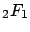,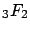or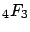hypergeometric functions. The method is identifying cases when the partial differential equations for Appell's functions imply hypergeometric ordinary differential equations for their univariate specializations. In general, ordinary differential equations for univariate specializations of Appell's functions have order at most 4.

As this work produces interesting but not immediately complete computations with a computer algebra package (like Maple or Mathematica), we try to formulate and demonstrate principles for electronic "preprint" or "article" publication of such computations, to be usable and perhaps developable further by other researchers.

Computing Dimension of Solution Spaces for Linear Functional Systems

Min Wu

Institute of Theoretical Computing, East China Normal University, Shanghai, China
mwu@sei.ecnu.edu.cn
A linear (partial) functional system is a mathematical abstraction of common properties of linear partial differential, difference operators or any mixture thereof. In this talk, we present an approach to determining dimension of solution spaces of linear functional systems. We introduce the notion of reflexive modules, which are naturally associated with reflexive systems that have the same solutions as the original system. We show that linear dimension of a linear functional system can be determined by Gröbner basis computation of reflexive modules over Ore algebras.

Evelyne Hubert 2008-05-26